is a square. With centres B and C and radius equal to the side of the square, circles are drawn to cut one another at E inside the square. What is the value of the angle  ?

#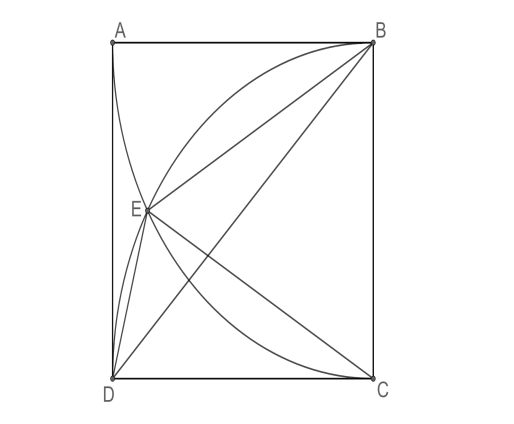1. A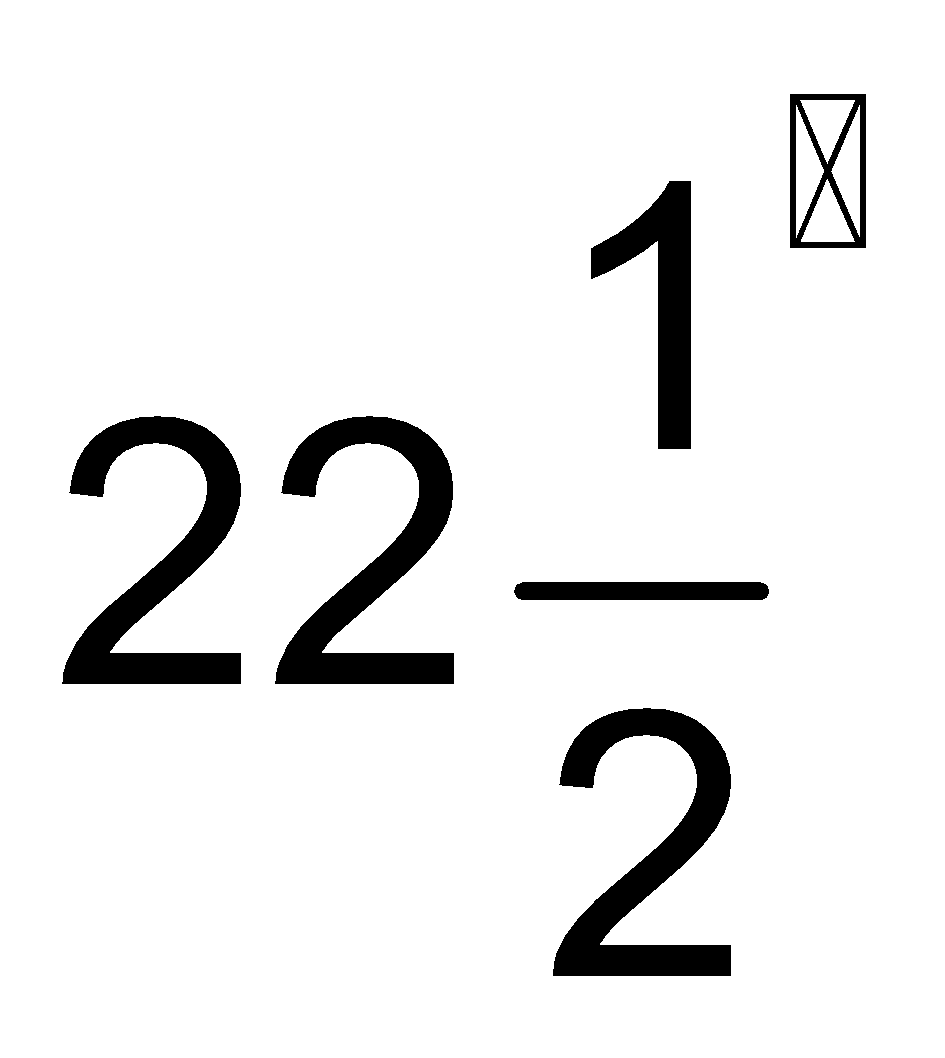2. B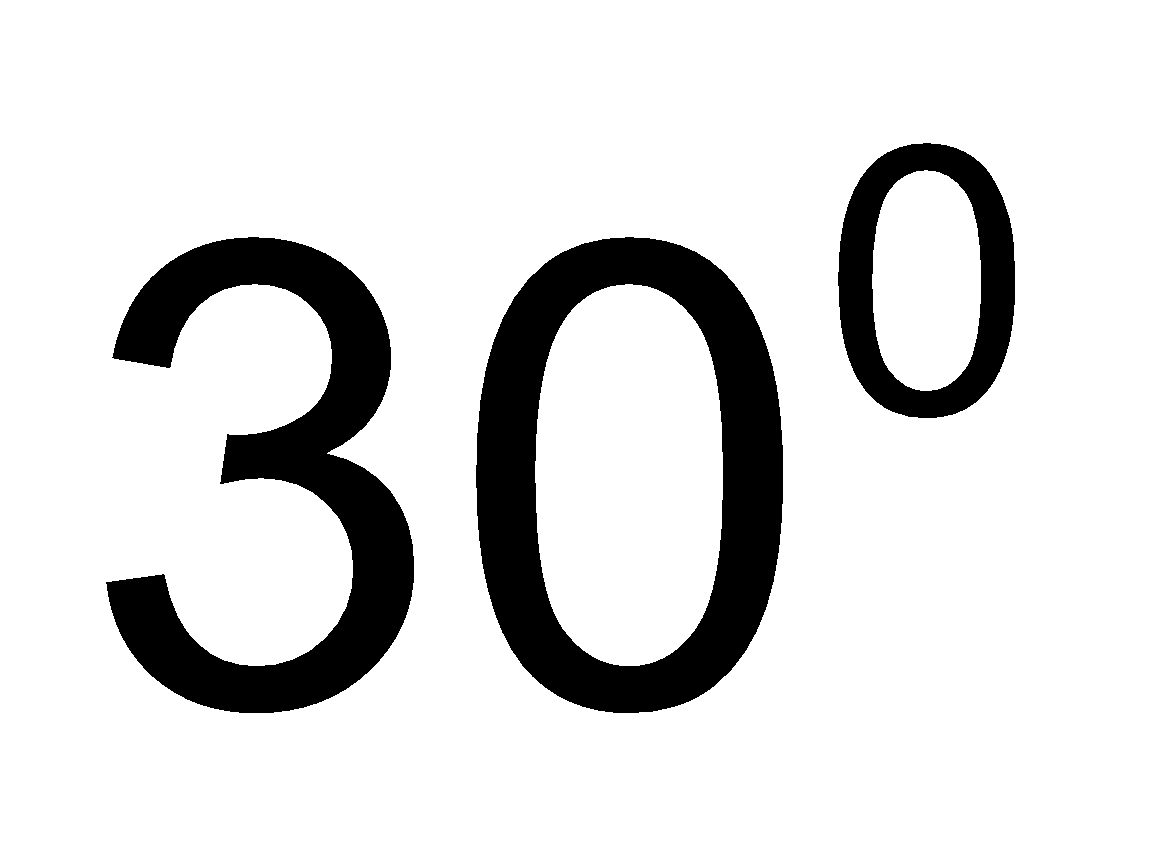3. C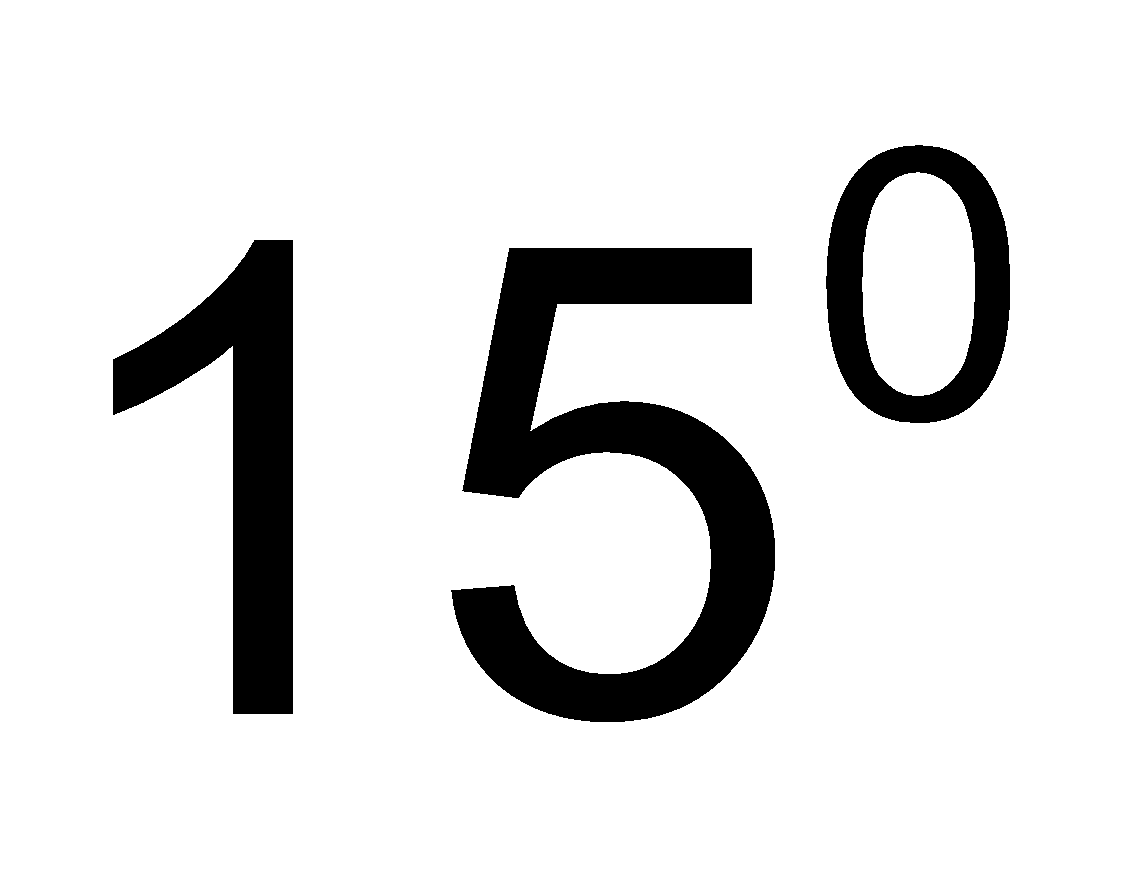4. D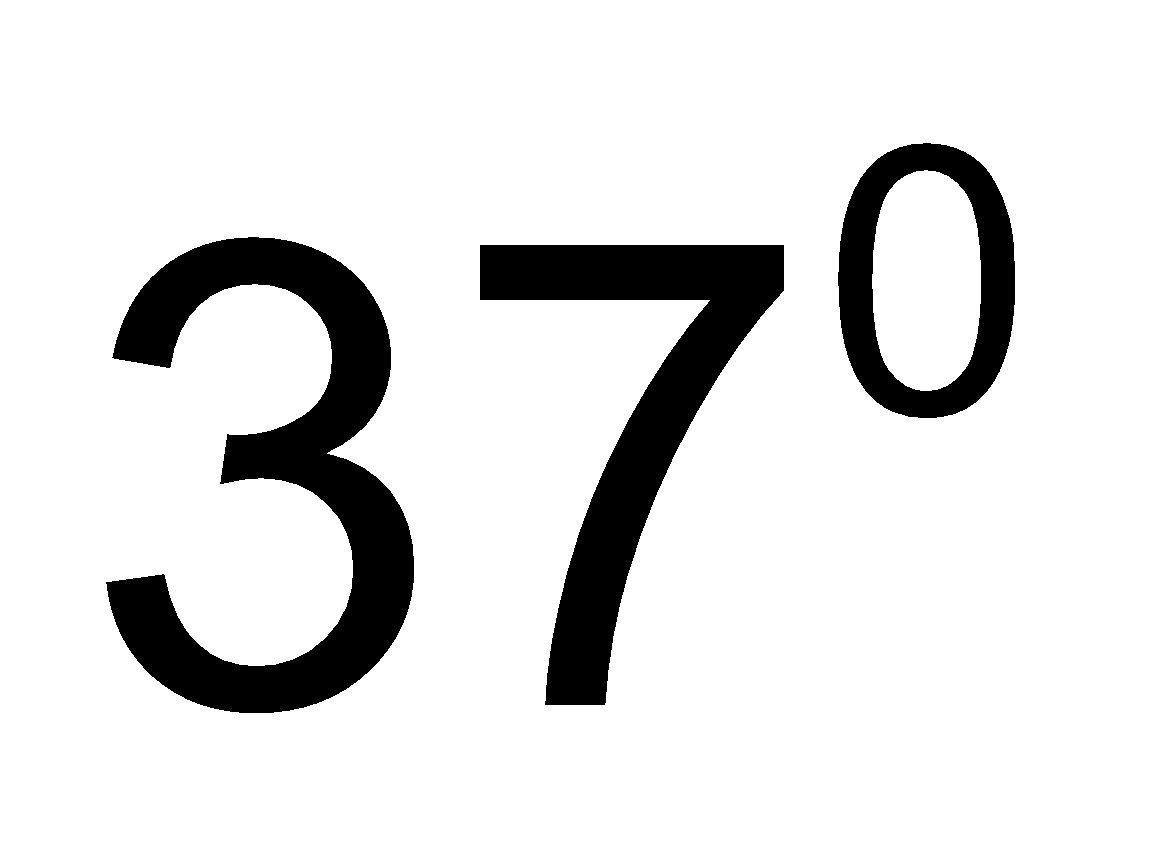Fill Out the Form for Expert Academic Guidance!l

+91

Live ClassesBooksTest SeriesSelf Learning

Verify OTP Code (required)

### Solution:

Let say side of square = Radius = a
Then BC = BE = CE  = a
Equilateral triangle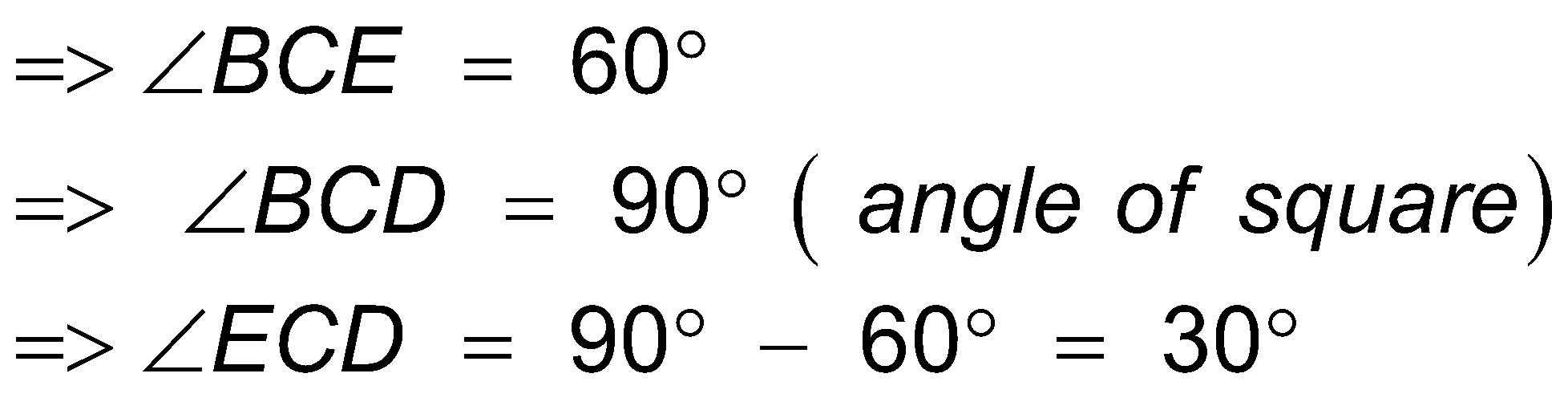now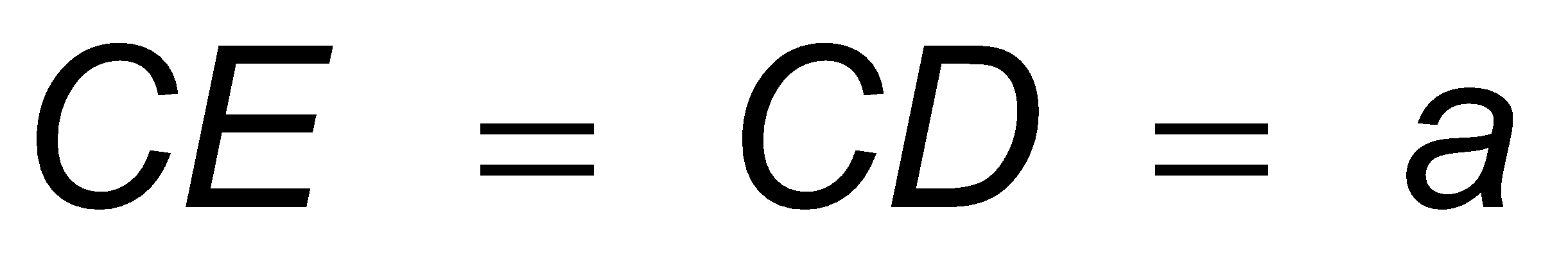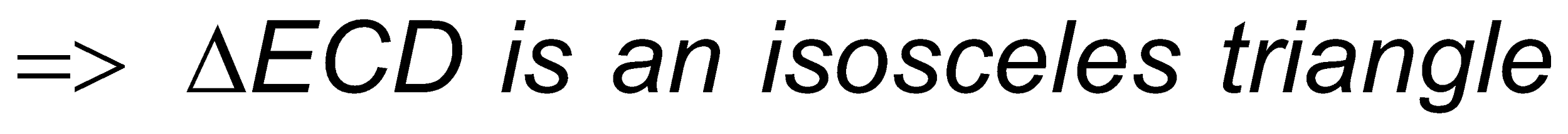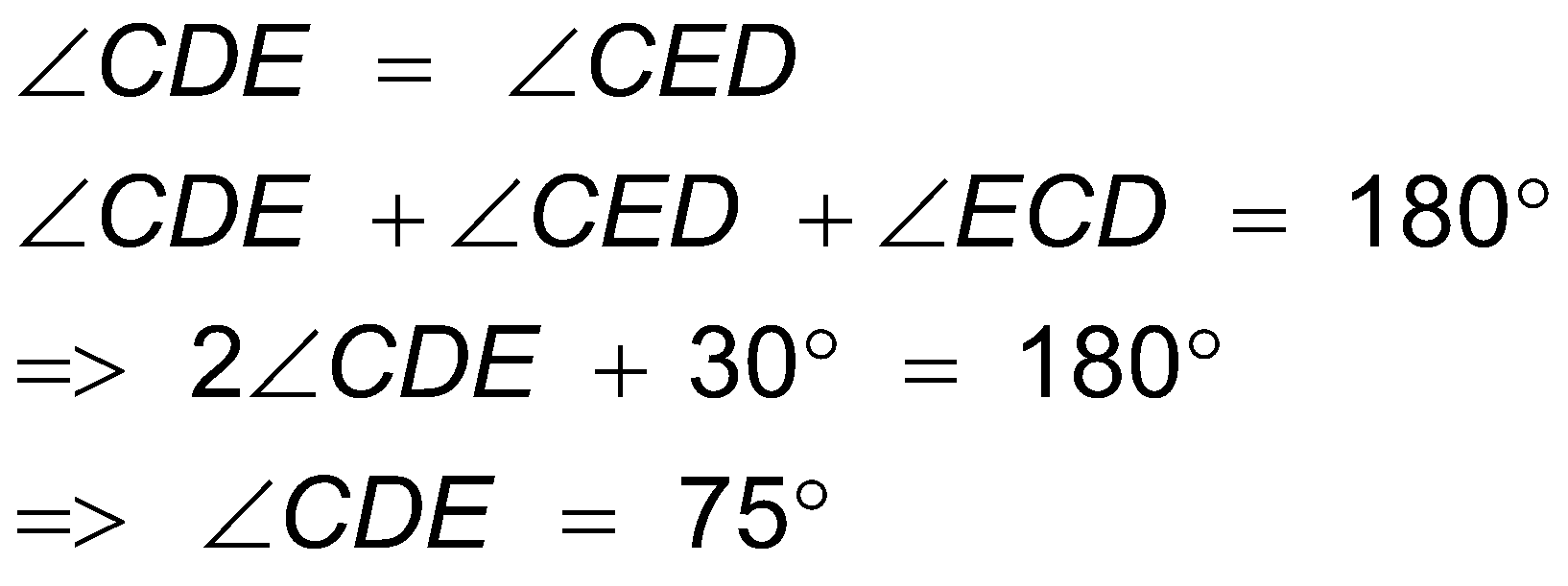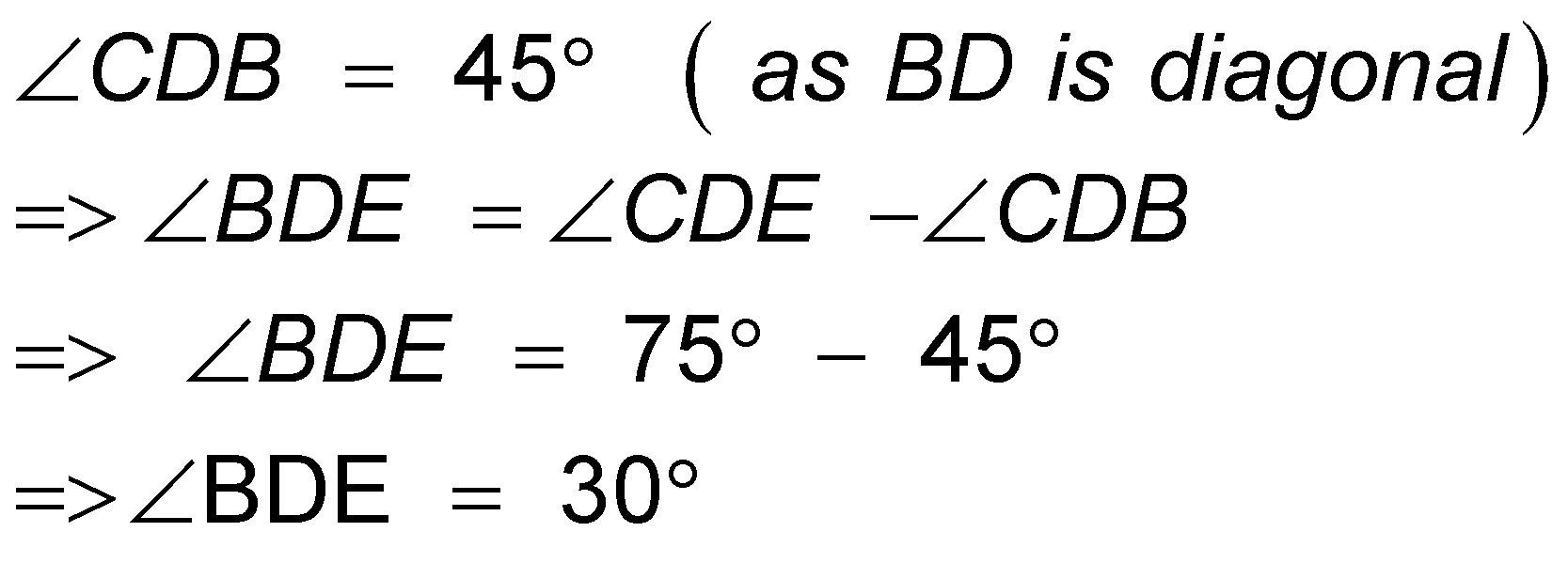So, option 2 is correct.

## Related content

 Area of Square Area of Isosceles Triangle Pythagoras Theorem Triangle Formula Perimeter of Triangle Formula Area Formulae Volume of Cone Formula Matrices and Determinants_mathematics Critical Points Solved Examples Type of relations_mathematics+91

Live ClassesBooksTest SeriesSelf Learning

Verify OTP Code (required)